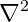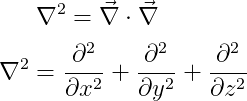# How to denote laplacian operator(∇²) in LaTeX?

Laplacian or Laplace operator is a differential operator, denoted by the square of dell operator(∇²).

In latex, the Laplacian operator is represented by \nabla^{2} command syntax.

\documentclass{article}
\begin{document}
$\nabla^{2}$
\end{document}

Output :And the Laplacian operator is expressed in the form of a dot product of two gradient operators. For example

\documentclass{article}
\begin{document}
$\nabla^{2}=\vec{\nabla}\cdot\vec{\nabla}$
$\nabla^{2}=\frac{\partial^2 }{\partial x^2}+\frac{\partial^2 }{\partial y^2}+\frac{\partial^2 }{\partial z^2}$
\end{document}

Output :The best practice is to install the physics package to identify the Laplacian operator in the best way because the physics package contains the predetermined \laplacian command to represent this operator.

\documentclass{article}
\usepackage{physics}
\begin{document}
$\laplacian$
$\laplacian{f}$
$\laplacian(f_{1}+f_{2})$
$\laplacian[f_{1}+f_{2}+\cdots+f_{n}]$
\end{document}

Output :In latex, nabla symbol of the laplacian operation cannot be in a bold format or a vector arrow mark above the symbol. Because this operator is a scalar operator.#### Md Jidan Mondal

LaTeX expert with over 10 years of experience in document preparation and typesetting. Specializes in creating professional documents, reports, and presentations using LaTeX.